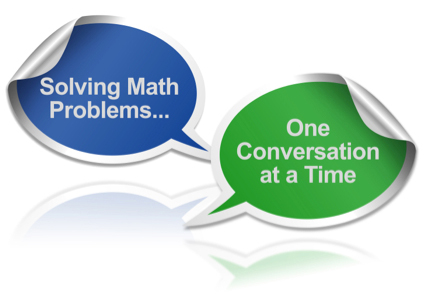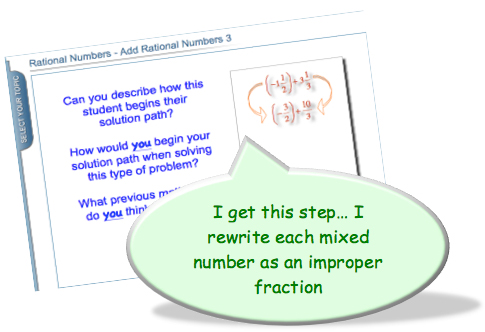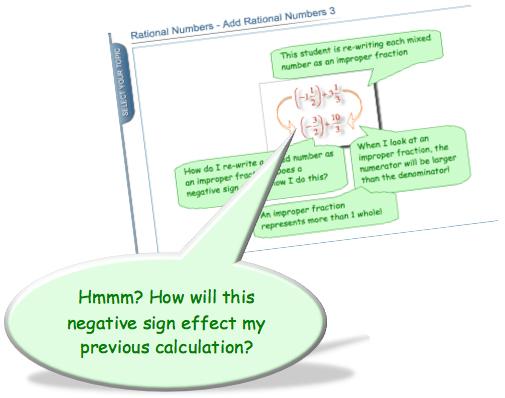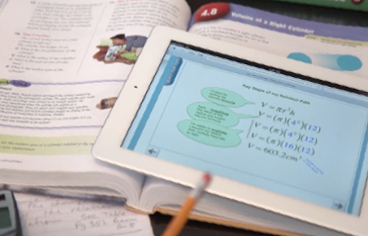Math homework can be challenging – especially when you have a page of problems that you do not yet understand how to solve. An important part of any learning process involves overcoming the challenges that can arise when trying to solve new problems.

Get It Guide Math Tutorials walk you step-by-step through the solution paths of math problems similar to those found in your homework assignments. We’ll help you determine the solution steps you understand and identify the solution steps still requiring additional help and support!

## How do I get started?

Look at the types of problems in your homework assignment (i.e. subtracting integers, multiplying rational numbers, solving 1-step equations)

Click on MATH TOPICS at the top of the web page and browse for the topic that matches your math problem.

Select the Slideshow Tutorial, a Worksheet or Video that matches the types of problems in your homework assignments.

## How should I work through a tutorial?

Get It Guide Math Tutorials will walk you step-by-step through a solution path using a series of questions similar to what your teacher could ask you in class.

After reading each question, it’s important to take some wait time (3-5 seconds) while you think of your response.

Remember, learning involves making errors, so don’t be afraid to think-aloud or jot down your answer… you might be surprised how much you actually  know!Once you’ve responded to a question, advance the tutorial and compare your response. Comparing your response will help you think about how you understand, explain and demonstrate a particular step within the solution path.

Work through the tutorial until you discover a step in the solution path where you feel you need help from your teacher.

## How do I keep my learning moving forward?

Your goal when working through a tutorial is trying to determine what you understand about a solution path, and more importantly… what you don’t understand.

• Identifying the steps in a solution path you understand will help build your confidence when solving math problems.
• Identifying the steps you do not understand will help you establish learning-oriented goals.
• Your learning-oriented goals form the questions you can ask your teacher for help with next math class!